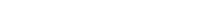# Math riddles Level 38 Answer with Hints and solutionsMath riddles game level 38 Answer with solution android game developed by Black games. Scroll Below to find details.

Math Riddles tests your IQ with mathematical puzzles. Challenge yourself with different levels of math puzzles and stretch the limits of your intelligence. Every IQ game is prepared with an approach of an IQ test. Improve your mathematics, challenge your brain with this game. There are many hard levels which you may not have solved then you can take help from here.

Math app level 38:

32=25

47=18

23=52

74=?Solution:

32 is written as 23 in  3rd row

25 is written as 52 in 3rd row

47 is written as 74 in 4th row

=> 18 will be written as 81 in 4th row

If there is any Doubt/discrepancy then please let me know in comments or you are unable to understand solution then I will surely help you.

### One thought on “Math Riddles App Level 38 Solution Android”

•December 6, 2019 at 7:50 pm